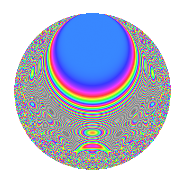# Properties

 Label 2013.2.aLevel 2013 Weight 2 Character orbit a Rep. character $$\chi_{2013}(1,\cdot)$$ Character field $$\Q$$ Dimension 99 Newforms 8 Sturm bound 496 Trace bound 7

# Related objects

## Defining parameters

 Level: $$N$$ = $$2013 = 3 \cdot 11 \cdot 61$$ Weight: $$k$$ = $$2$$ Character orbit: $$[\chi]$$ = 2013.a (trivial) Character field: $$\Q$$ Newforms: $$8$$ Sturm bound: $$496$$ Trace bound: $$7$$ Distinguishing $$T_p$$: $$2$$

## Dimensions

The following table gives the dimensions of various subspaces of $$M_{2}(\Gamma_0(2013))$$.

Total New Old
Modular forms 252 99 153
Cusp forms 245 99 146
Eisenstein series 7 0 7

The following table gives the dimensions of the cuspidal new subspaces with specified eigenvalues for the Atkin-Lehner operators and the Fricke involution.

$$3$$$$11$$$$61$$FrickeDim.
$$+$$$$+$$$$+$$$$+$$$$12$$
$$+$$$$+$$$$-$$$$-$$$$14$$
$$+$$$$-$$$$+$$$$-$$$$13$$
$$+$$$$-$$$$-$$$$+$$$$11$$
$$-$$$$+$$$$+$$$$-$$$$13$$
$$-$$$$+$$$$-$$$$+$$$$11$$
$$-$$$$-$$$$+$$$$+$$$$12$$
$$-$$$$-$$$$-$$$$-$$$$13$$
Plus space$$+$$$$46$$
Minus space$$-$$$$53$$

## Trace form

 $$99q$$ $$\mathstrut -\mathstrut 3q^{2}$$ $$\mathstrut -\mathstrut q^{3}$$ $$\mathstrut +\mathstrut 93q^{4}$$ $$\mathstrut -\mathstrut 6q^{5}$$ $$\mathstrut -\mathstrut 3q^{6}$$ $$\mathstrut -\mathstrut 8q^{7}$$ $$\mathstrut +\mathstrut 9q^{8}$$ $$\mathstrut +\mathstrut 99q^{9}$$ $$\mathstrut +\mathstrut O(q^{10})$$ $$99q$$ $$\mathstrut -\mathstrut 3q^{2}$$ $$\mathstrut -\mathstrut q^{3}$$ $$\mathstrut +\mathstrut 93q^{4}$$ $$\mathstrut -\mathstrut 6q^{5}$$ $$\mathstrut -\mathstrut 3q^{6}$$ $$\mathstrut -\mathstrut 8q^{7}$$ $$\mathstrut +\mathstrut 9q^{8}$$ $$\mathstrut +\mathstrut 99q^{9}$$ $$\mathstrut +\mathstrut 6q^{10}$$ $$\mathstrut -\mathstrut q^{11}$$ $$\mathstrut -\mathstrut 7q^{12}$$ $$\mathstrut -\mathstrut 14q^{13}$$ $$\mathstrut +\mathstrut 16q^{14}$$ $$\mathstrut -\mathstrut 6q^{15}$$ $$\mathstrut +\mathstrut 85q^{16}$$ $$\mathstrut -\mathstrut 10q^{17}$$ $$\mathstrut -\mathstrut 3q^{18}$$ $$\mathstrut -\mathstrut 12q^{19}$$ $$\mathstrut -\mathstrut 2q^{20}$$ $$\mathstrut -\mathstrut 8q^{21}$$ $$\mathstrut -\mathstrut 3q^{22}$$ $$\mathstrut -\mathstrut 15q^{24}$$ $$\mathstrut +\mathstrut 85q^{25}$$ $$\mathstrut -\mathstrut 26q^{26}$$ $$\mathstrut -\mathstrut q^{27}$$ $$\mathstrut +\mathstrut 10q^{29}$$ $$\mathstrut +\mathstrut 14q^{30}$$ $$\mathstrut +\mathstrut 8q^{31}$$ $$\mathstrut -\mathstrut 15q^{32}$$ $$\mathstrut +\mathstrut 3q^{33}$$ $$\mathstrut +\mathstrut 2q^{34}$$ $$\mathstrut +\mathstrut 32q^{35}$$ $$\mathstrut +\mathstrut 93q^{36}$$ $$\mathstrut -\mathstrut 14q^{37}$$ $$\mathstrut -\mathstrut 20q^{38}$$ $$\mathstrut -\mathstrut 14q^{39}$$ $$\mathstrut +\mathstrut 30q^{40}$$ $$\mathstrut -\mathstrut 10q^{41}$$ $$\mathstrut +\mathstrut 24q^{42}$$ $$\mathstrut -\mathstrut 28q^{43}$$ $$\mathstrut +\mathstrut 9q^{44}$$ $$\mathstrut -\mathstrut 6q^{45}$$ $$\mathstrut -\mathstrut 16q^{46}$$ $$\mathstrut +\mathstrut 8q^{47}$$ $$\mathstrut +\mathstrut q^{48}$$ $$\mathstrut +\mathstrut 67q^{49}$$ $$\mathstrut -\mathstrut 69q^{50}$$ $$\mathstrut -\mathstrut 18q^{51}$$ $$\mathstrut -\mathstrut 58q^{52}$$ $$\mathstrut -\mathstrut 22q^{53}$$ $$\mathstrut -\mathstrut 3q^{54}$$ $$\mathstrut +\mathstrut 10q^{55}$$ $$\mathstrut +\mathstrut 32q^{56}$$ $$\mathstrut -\mathstrut 20q^{57}$$ $$\mathstrut +\mathstrut 6q^{58}$$ $$\mathstrut -\mathstrut 4q^{59}$$ $$\mathstrut -\mathstrut 2q^{60}$$ $$\mathstrut -\mathstrut q^{61}$$ $$\mathstrut -\mathstrut 24q^{62}$$ $$\mathstrut -\mathstrut 8q^{63}$$ $$\mathstrut +\mathstrut 29q^{64}$$ $$\mathstrut -\mathstrut 68q^{65}$$ $$\mathstrut -\mathstrut 3q^{66}$$ $$\mathstrut +\mathstrut 4q^{67}$$ $$\mathstrut -\mathstrut 38q^{68}$$ $$\mathstrut +\mathstrut 8q^{69}$$ $$\mathstrut +\mathstrut 8q^{71}$$ $$\mathstrut +\mathstrut 9q^{72}$$ $$\mathstrut -\mathstrut 10q^{73}$$ $$\mathstrut -\mathstrut 50q^{74}$$ $$\mathstrut -\mathstrut 31q^{75}$$ $$\mathstrut +\mathstrut 36q^{76}$$ $$\mathstrut -\mathstrut 8q^{77}$$ $$\mathstrut -\mathstrut 2q^{78}$$ $$\mathstrut -\mathstrut 40q^{79}$$ $$\mathstrut -\mathstrut 18q^{80}$$ $$\mathstrut +\mathstrut 99q^{81}$$ $$\mathstrut -\mathstrut 22q^{82}$$ $$\mathstrut +\mathstrut 4q^{83}$$ $$\mathstrut -\mathstrut 24q^{84}$$ $$\mathstrut -\mathstrut 36q^{85}$$ $$\mathstrut +\mathstrut 52q^{86}$$ $$\mathstrut -\mathstrut 6q^{87}$$ $$\mathstrut -\mathstrut 15q^{88}$$ $$\mathstrut -\mathstrut 26q^{89}$$ $$\mathstrut +\mathstrut 6q^{90}$$ $$\mathstrut +\mathstrut 16q^{91}$$ $$\mathstrut -\mathstrut 72q^{92}$$ $$\mathstrut -\mathstrut 24q^{93}$$ $$\mathstrut -\mathstrut 16q^{94}$$ $$\mathstrut +\mathstrut 64q^{95}$$ $$\mathstrut -\mathstrut 23q^{96}$$ $$\mathstrut -\mathstrut 66q^{97}$$ $$\mathstrut -\mathstrut 59q^{98}$$ $$\mathstrut -\mathstrut q^{99}$$ $$\mathstrut +\mathstrut O(q^{100})$$

## Decomposition of $$S_{2}^{\mathrm{new}}(\Gamma_0(2013))$$ into irreducible Hecke orbits

Label Dim. $$A$$ Field CM Traces A-L signs $q$-expansion
$$a_2$$ $$a_3$$ $$a_5$$ $$a_7$$ 3 11 61
2013.2.a.a $$11$$ $$16.074$$ $$\mathbb{Q}[x]/(x^{11} - \cdots)$$ None $$-4$$ $$11$$ $$-13$$ $$-5$$ $$-$$ $$+$$ $$-$$ $$q-\beta _{1}q^{2}+q^{3}+(1+\beta _{5}+\beta _{6})q^{4}+(-1+\cdots)q^{5}+\cdots$$
2013.2.a.b $$11$$ $$16.074$$ $$\mathbb{Q}[x]/(x^{11} - \cdots)$$ None $$-2$$ $$-11$$ $$-1$$ $$-11$$ $$+$$ $$-$$ $$-$$ $$q-\beta _{1}q^{2}-q^{3}+(1+\beta _{2})q^{4}+\beta _{8}q^{5}+\cdots$$
2013.2.a.c $$12$$ $$16.074$$ $$\mathbb{Q}[x]/(x^{12} - \cdots)$$ None $$-7$$ $$12$$ $$-7$$ $$-15$$ $$-$$ $$-$$ $$+$$ $$q+(-1+\beta _{1})q^{2}+q^{3}+(2-\beta _{1}+\beta _{2}+\cdots)q^{4}+\cdots$$
2013.2.a.d $$12$$ $$16.074$$ $$\mathbb{Q}[x]/(x^{12} - \cdots)$$ None $$1$$ $$-12$$ $$-3$$ $$-9$$ $$+$$ $$+$$ $$+$$ $$q+\beta _{1}q^{2}-q^{3}+(1+\beta _{2})q^{4}-\beta _{3}q^{5}+\cdots$$
2013.2.a.e $$13$$ $$16.074$$ $$\mathbb{Q}[x]/(x^{13} - \cdots)$$ None $$2$$ $$-13$$ $$3$$ $$11$$ $$+$$ $$-$$ $$+$$ $$q+\beta _{1}q^{2}-q^{3}+(1+\beta _{2})q^{4}-\beta _{8}q^{5}+\cdots$$
2013.2.a.f $$13$$ $$16.074$$ $$\mathbb{Q}[x]/(x^{13} - \cdots)$$ None $$4$$ $$13$$ $$7$$ $$5$$ $$-$$ $$+$$ $$+$$ $$q+\beta _{1}q^{2}+q^{3}+(1+\beta _{1}+\beta _{2})q^{4}+(1+\cdots)q^{5}+\cdots$$
2013.2.a.g $$13$$ $$16.074$$ $$\mathbb{Q}[x]/(x^{13} - \cdots)$$ None $$4$$ $$13$$ $$7$$ $$7$$ $$-$$ $$-$$ $$-$$ $$q+\beta _{1}q^{2}+q^{3}+(1+\beta _{2})q^{4}+(1+\beta _{5}+\cdots)q^{5}+\cdots$$
2013.2.a.h $$14$$ $$16.074$$ $$\mathbb{Q}[x]/(x^{14} - \cdots)$$ None $$-1$$ $$-14$$ $$1$$ $$9$$ $$+$$ $$+$$ $$-$$ $$q-\beta _{1}q^{2}-q^{3}+(1+\beta _{2})q^{4}+\beta _{10}q^{5}+\cdots$$

## Decomposition of $$S_{2}^{\mathrm{old}}(\Gamma_0(2013))$$ into lower level spaces

$$S_{2}^{\mathrm{old}}(\Gamma_0(2013)) \cong$$ $$S_{2}^{\mathrm{new}}(\Gamma_0(11))$$$$^{\oplus 4}$$$$\oplus$$$$S_{2}^{\mathrm{new}}(\Gamma_0(33))$$$$^{\oplus 2}$$$$\oplus$$$$S_{2}^{\mathrm{new}}(\Gamma_0(61))$$$$^{\oplus 4}$$$$\oplus$$$$S_{2}^{\mathrm{new}}(\Gamma_0(183))$$$$^{\oplus 2}$$$$\oplus$$$$S_{2}^{\mathrm{new}}(\Gamma_0(671))$$$$^{\oplus 2}$$